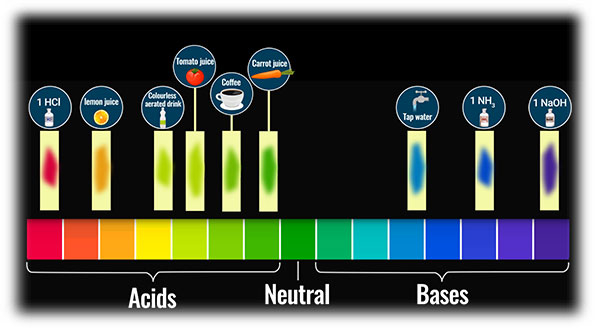# (a) What do you think will be the pH in the stomach of a person suffering from indigestion: less than 7 or more than 7?(b) What do you think will be the pH of an antacid solution: less than 7 or more than 7?(c) How does an antacid work?(d) Name two common antacids.

(a) Indigestion occurs due to the production of excess hydrochloric acid in the stomach caused due to overeating, therefore the pH should be less than 7.

(b)  Antacid solution is a base so the pH will be greater than 7.

(c)  Antacids are bases that neutralize excess acid formed inside the stomach due to indigestion.

(d) Magnesium hydroxide and Sodium hydrogen carbonate are two antacids used.

[pH Scale is used to measure acidity or alkalinity of water-soluble substances (pH stands for 'potential of Hydrogen'). It measures H+ concentration in a solution.
• The pH scale ranges from 0 to 14.
• A pH less than 7 is acidic in nature.
• A pH of 7 is neutral.
• A pH greater than 7 is basic in nature.

(Note: The pH of a solution is a measure of the molar concentration of hydrogen ions in the solution and as such is a measure of the acidity or basicity of the solution.

The letters pH stand for "power of hydrogen" and the numerical value for pH is just the negative of the power of 10 of the molar concentration of H+ ions.

To calculate the pH of an aqueous solution you need to know the concentration of the hydronium ion in moles per litre (molarity).

The pH is then calculated using the expression: pH = - log [H3O+]. )]

Updated on: 10-Oct-2022

49 Views